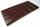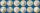# Circle

How big is area of circle if its circumference is 80.6 cm?

Result

S =  517 cm2

#### Solution:

$o = 2 \pi r = 80.6 \ \\ S = \pi r^2 \ \\ \ \\ S = \pi \left( \dfrac{ 80.6}{2\pi} \right)^2 = 517 \ cm^2$

Leave us a comment of this math problem and its solution (i.e. if it is still somewhat unclear...):Be the first to comment!## Next similar math problems:

1. Square gridSquare grid consists of a square with sides of length 1 cm. Draw in it at least three different patterns such that each had a content of 6 cm2 and circumference 12 cm and that their sides is in square grid.
2. WindowsCalculate the area of masonry to build wall with dimensions of 9 m × 4 m with 4 windows of size 64 cm × 64 cm.
3. PlumberPlumber had to cut the metal strip with dimensions 380 cm and 60 cm to the largest squares so that no waste. Calculate the length of the sides of a square. How many squares cut it?
4. RectanglesCalculate how many squares/rectangles of size 4×3 cm can be cut from a sheet of paper of 36 cm × 32 cm?
5. ChocholateTable of chocolate is divided into squares on its surface. Lengthwise has 15 squares and widthwise 19 squares. We must chocolate broke into individual squares. How many times we have broke it to get only individual squares? It is not permitted to break
6. RainfallAnnual rainfall in our country are an average of 797 mm. How many m3 of water rains on average per hectare?
7. Two squaresTwo squares whose sides are in the ratio 5:2 have sum of its perimeters 73 cm. Calculate the sum of area this two squares.
8. PresentGift box has rectangular shape with dimensions of 8×8×3 cm. Miloslav wants to cover with square paper with sides of 18 cm. How much paper left him?
9. Maximum area of rhombusCalculate the interior angles at which equilateral rhombus has a maximum area.
10. Mr. ZucchiniMr. Zucchini had a rectangular garden whose perimeter is 28 meters. Content area of the garden filled just four square beds, whose dimensions in meters are expressed in whole numbers. Determine what size could have a garden. Find all the possibilities and
11. GardenTrapezoid garden has parallel sides 19 m and 24 m. Its area is 193.5 square meters. What is the width of the garden?
12. CoinsHarvey had saved up a number of 2-euro coins. He stored coins in a single layer in a square. Left 6 coins. When he make square, which has one more row, missing 35 coins. How many euros he have?
13. Hexagon - MOThe picture shows the ABCD square, the EFGD square and the HIJD rectangle. Points J and G lie on the side CD and is true |DJ|
14. Area of trapezoidThe trapezoid bases are and 7 dm and 11 cm. His height is 4 cm. Calculate the area of trapezoid.
15. The potThe pot is in 1/3 filled with water. Bottom of the pot has an area of ​​329 cm2. How many centimeters rises water level in the pot after add 1.2 liters of water?
16. PoolMr. Peter build a pool shape of a four-sided prism with rhombus base in the garden. Base edge length is 8 m, distance of the opposite walls of the pool is 7 m. Estimated depth is 144 cm. How many hectoliters of water consume Mr. Peter to fill the pool?
17. Plan of the villagePlan of the municipality in 1:1000 scale has plotted garden with dimensions 25 mm and 28 mm. Determine the area of gardens in ares in reality.